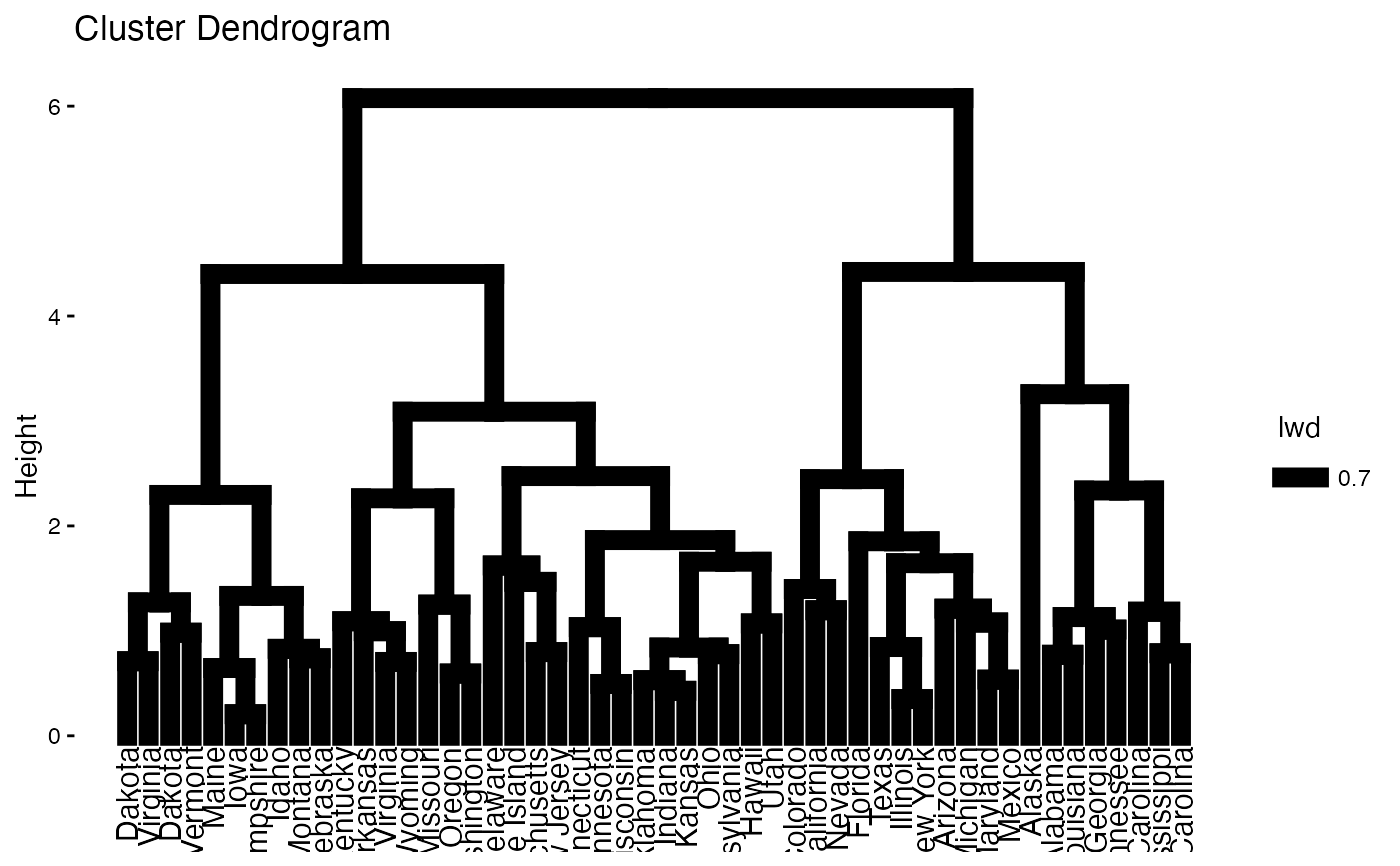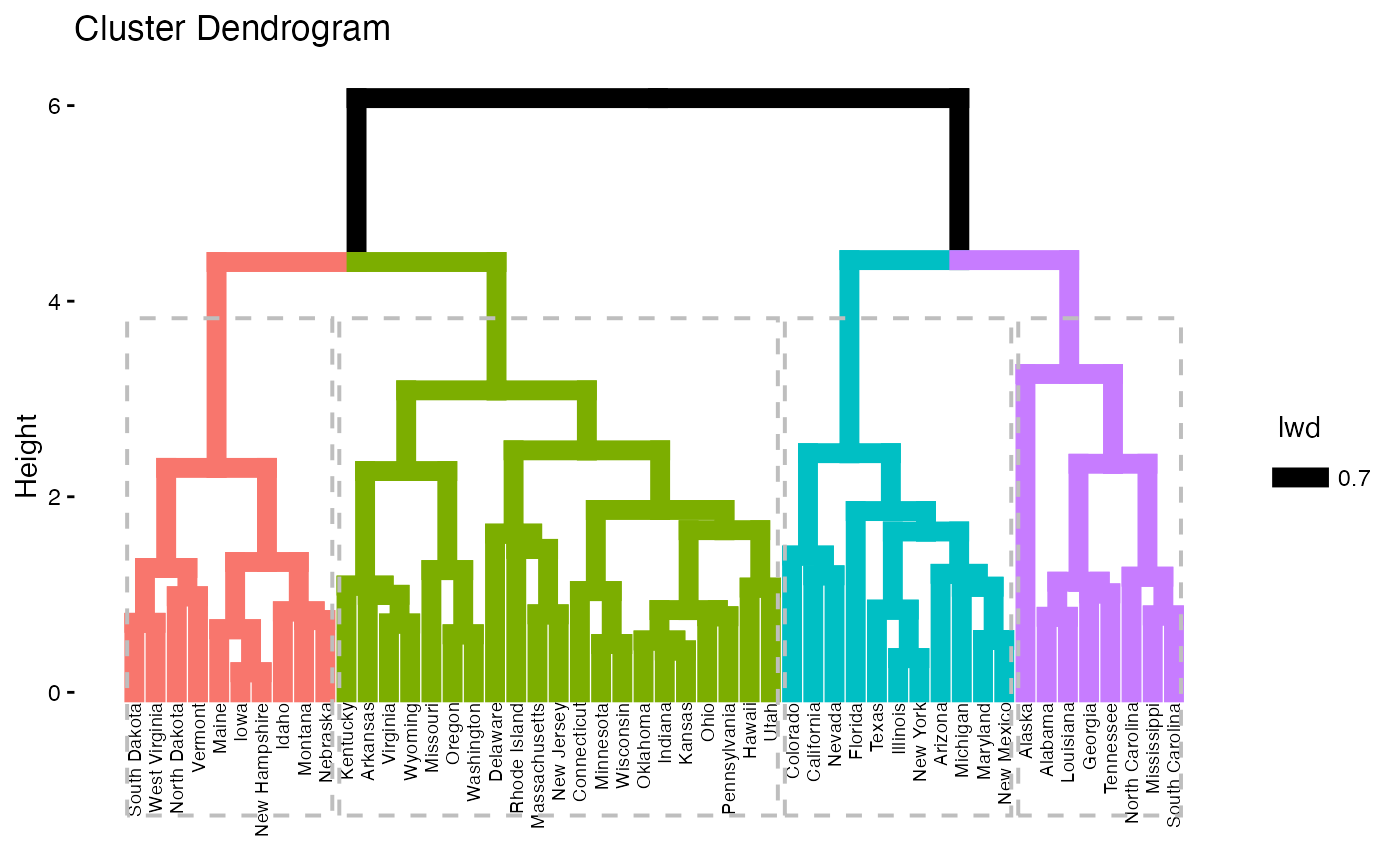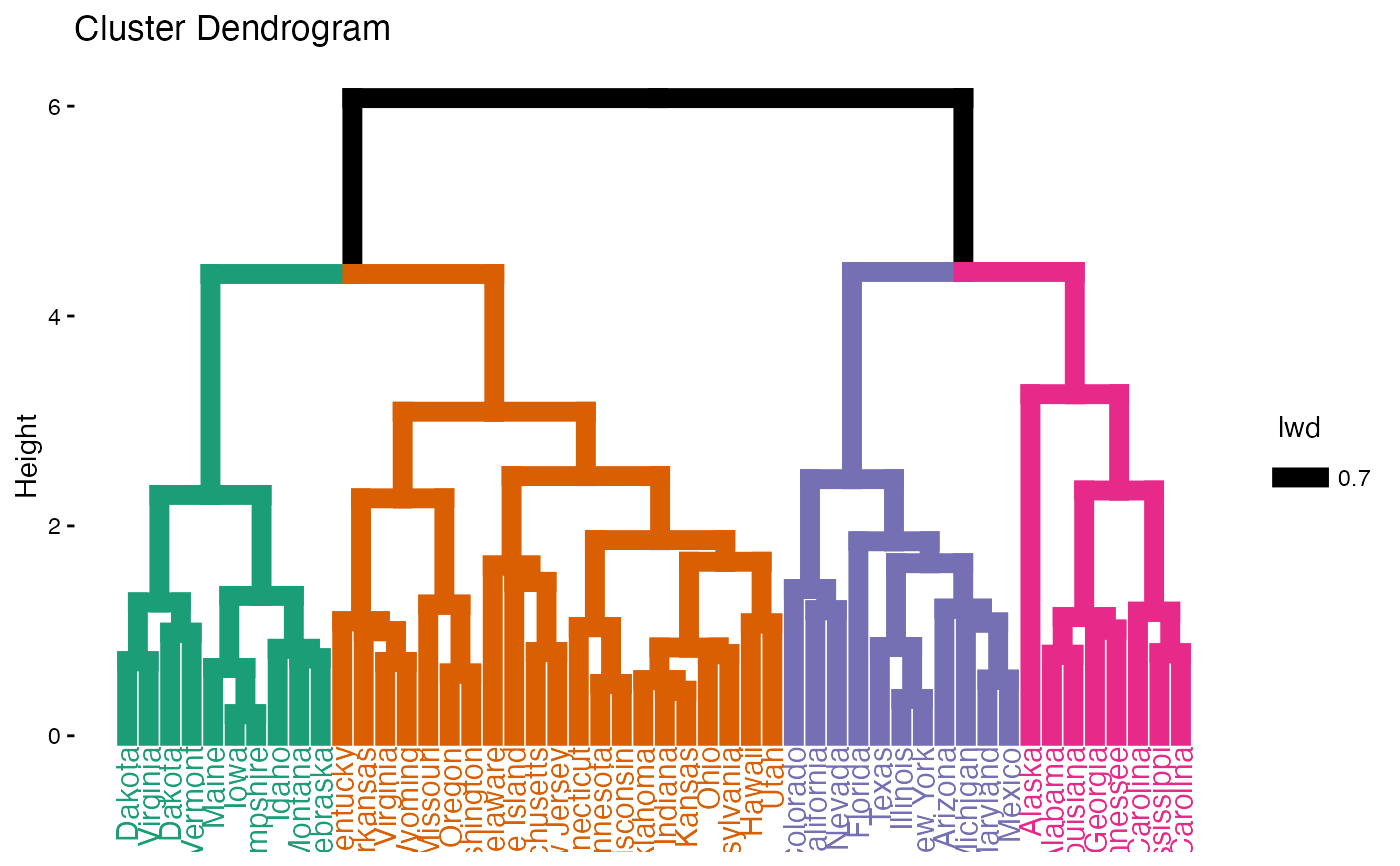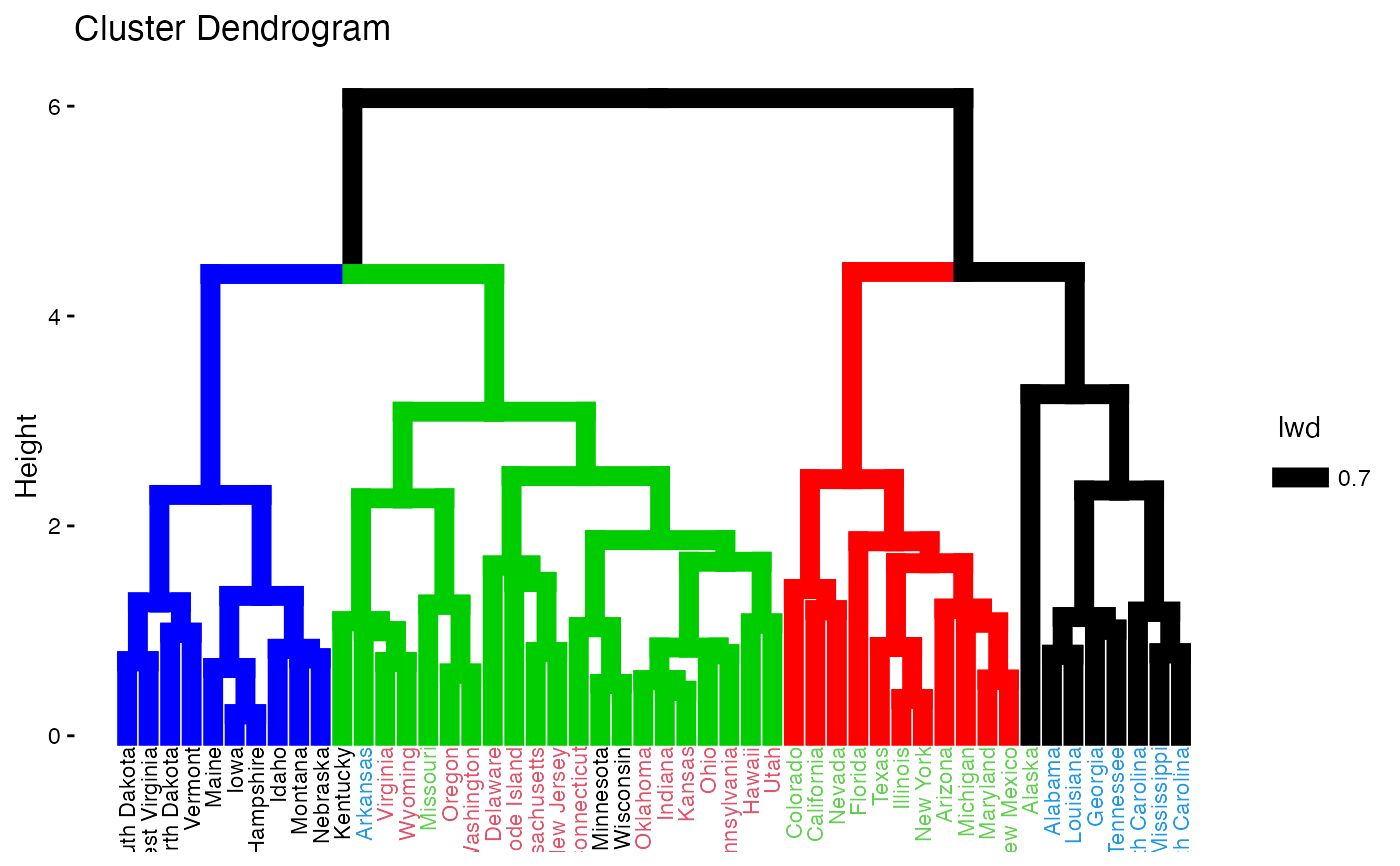Draws easily beautiful dendrograms using either R base plot or ggplot2. Provides also an option for drawing a circular dendrogram and phylogenic trees.

fviz_dend(x, k = NULL, h = NULL, k_colors = NULL, palette = NULL,
show_labels = TRUE, color_labels_by_k = TRUE, label_cols = NULL,
labels_track_height = NULL, repel = FALSE, lwd = 0.7,
type = c("rectangle", "circular", "phylogenic"),
phylo_layout = "layout.auto", rect = FALSE, rect_border = "gray",
rect_lty = 2, rect_fill = FALSE, lower_rect, horiz = FALSE,
cex = 0.8, main = "Cluster Dendrogram", xlab = "",
ylab = "Height", sub = NULL, ggtheme = theme_classic(), ...)

## Arguments

x an object of class dendrogram, hclust, agnes, diana, hcut, hkmeans or HCPC (FactoMineR). the number of groups for cutting the tree. a numeric value. Cut the dendrogram by cutting at height h. (k overrides h) a vector containing colors to be used for the groups. It should contains k number of colors. Allowed values include also "grey" for grey color palettes; brewer palettes e.g. "RdBu", "Blues", ...; and scientific journal palettes from ggsci R package, e.g.: "npg", "aaas", "lancet", "jco", "ucscgb", "uchicago", "simpsons" and "rickandmorty". a logical value. If TRUE, leaf labels are shown. Default value is TRUE. logical value. If TRUE, labels are colored automatically by group when k != NULL. a vector containing the colors for labels. a positive numeric value for adjusting the room for the labels. Used only when type = "rectangle". logical value. Use repel = TRUE to avoid label overplotting when type = "phylogenic". a numeric value specifying branches and rectangle line width. type of plot. Allowed values are one of "rectangle", "triangle", "circular", "phylogenic". the layout to be used for phylogenic trees. Default value is "layout.auto". Allowed values include: layout.auto, layout_with_drl, layout_as_tree, layout.gem, layout.mds and layout_with_lgl. logical value specifying whether to add a rectangle around groups. Used only when k != NULL. border color and line type for rectangles. a logical value. If TRUE, fill the rectangle. a value of how low should the lower part of the rectangle around clusters. Ignored when rect = FALSE. a logical value. If TRUE, an horizontal dendrogram is drawn. size of labels main and axis titles Plot subtitle. If NULL, the method used hierarchical clustering is shown. To remove the subtitle use sub = "". function, ggplot2 theme name. Default value is theme_classic(). Allowed values include ggplot2 official themes: theme_gray(), theme_bw(), theme_minimal(), theme_classic(), theme_void(), .... other arguments to be passed to the function plot.dendrogram()

## Value

an object of class fviz_dend which is a ggplot with the attributes "dendrogram" accessible using attr(x, "dendrogram"), where x is the result of fviz_dend().

## Examples

# \donttest{
# Load and scale the data
data(USArrests)
df <- scale(USArrests)

# Hierarchical clustering
res.hc <- hclust(dist(df))

# Default plot
fviz_dend(res.hc)# Cut the tree
fviz_dend(res.hc, cex = 0.5, k = 4, color_labels_by_k = TRUE)# Don't color labels, add rectangles
fviz_dend(res.hc, cex = 0.5, k = 4,
color_labels_by_k = FALSE, rect = TRUE)# Change the color of tree using black color for all groups
# Change rectangle border colors
fviz_dend(res.hc, rect = TRUE, k_colors ="black",
rect_border = 2:5, rect_lty = 1)# Customized color for groups
fviz_dend(res.hc, k = 4,
k_colors = c("#1B9E77", "#D95F02", "#7570B3", "#E7298A"))# Color labels using k-means clusters
km.clust <- kmeans(df, 4)$cluster fviz_dend(res.hc, k = 4, k_colors = c("blue", "green3", "red", "black"), label_cols = km.clust[res.hc$order], cex = 0.6)# }